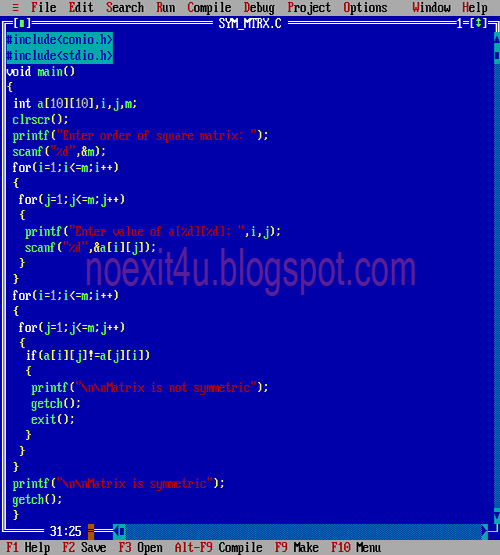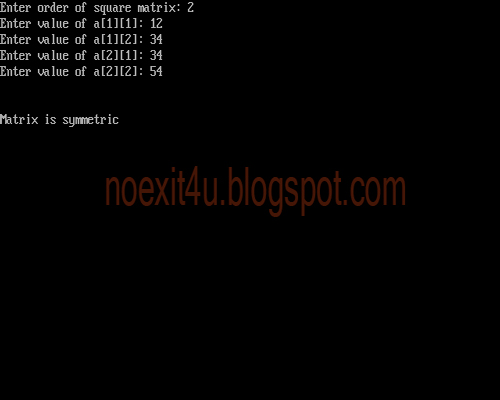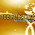Wednesday, 13 February 2013

C PROGRAM: TO CHECK WHETHER A MATRIX IS SYMMETRIC OR NOT

CODING:
#include<conio.h>
#include<stdio.h>
void main()
{
int a,i,j,m;
clrscr();
printf("Enter order of square matrix: ");
scanf("%d",&m);
for(i=1;i<=m;i++)
{
for(j=1;j<=m;j++)
{
printf("Enter value of a[%d][%d]: ",i,j);
scanf("%d",&a[i][j]);
}
}
for(i=1;i<=m;i++)
{
for(j=1;j<=m;j++)
{
if(a[i][j]!=a[j][i])
{
printf("\n\nMatrix is not symmetric");
getch();
exit(0);
}
}
}
printf("\n\nMatrix is symmetric");
getch();
}CODINGOUTPUT

For any query or suggestion please comment below...

1.thanks, really helped

2.correction required!
change exit() to exit(0)

3.Thanks .. here the algorithm implemented in above program.
1. Find transpose matrix of inputMatrix and store it in transposeMatrix.
Check this C program to find transpose matrix
2. Compare inputMatrix and transposeMatric.
Check this C program to compare two matrix
3. If both matrices are equal then inputMatrix is symmetric matrix otherwise not a symmetric matrix

4.5.1.